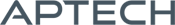# Linear Regression MT

Linear Regression MT

Availability: Out of stock

The Linear Regression MT application module is a set of procedures for estimating single equations or a simultaneous system of equations. It allows constraints on coefficients, calculates het-con standard errors, and includes two-stage least squares,...
Overview

## Linear Regression MT

The Linear Regression MT application module is a set of procedures for estimating single equations or a simultaneous system of equations. It allows constraints on coefficients, calculates het-con standard errors, and includes two-stage least squares, three-stage least squares, and seemingly unrelated regression. It is thread-safe and takes advantage of structures found in later versions of GAUSS.

Platform: Windows, Mac, and Linux.

Requirements: GAUSS/GAUSS Engine/GAUSS Light v8.0 or higher.

Features

## Features

• Calculates heteroskedastic-consistent standard errors, and performs both influence and collinearity diagnostics inside the ordinary least squares routine (OLS)
• All regression procedures can be run at a specified data range
• Performs multiple linear hypothesis testing with any form
• Estimates regressions with linear restrictions
• Accommodates large data sets with multiple variables
• Stores all important test statistics and estimated coefficients in an efficient manner
• Both three-stage least squares and seemingly unrelated regression can be estimated iteratively
• Thorough Documentation
• The comprehensive user's guide includes both a well-written tutorial and an informative reference section. Additional topics are included to enrich the usage of the procedures. These include:
• Joint confidence region for beta estimates
• Tests for heteroskedasticity
• Tests of structural change
• Using ordinary least squares to estimate a translog cost function
• Using seemingly unrelated regression to estimate a system of cost share equations
• Using three-stage least squares to estimate Klein's Model I

Product Inquiry

4 + 3 = enter the result ex:(3 + 2 = 5)

﻿